Related Articles

# Class 11 RD Sharma Solutions – Chapter 6 Graphs of Trigonometric Functions – Exercise 6.1

• Last Updated : 30 Apr, 2021

### (i) y = 2 sin 2x

Solution:

To obtain this graph y = 2 sin 2x,

Attention reader! All those who say programming isn't for kids, just haven't met the right mentors yet. Join the  Demo Class for First Step to Coding Coursespecifically designed for students of class 8 to 12.

The students will get to learn more about the world of programming in these free classes which will definitely help them in making a wise career choice in the future.

First we draw the graph of y = sin x and then divide the x-coordinates of the points where it crosses x-axis by 2.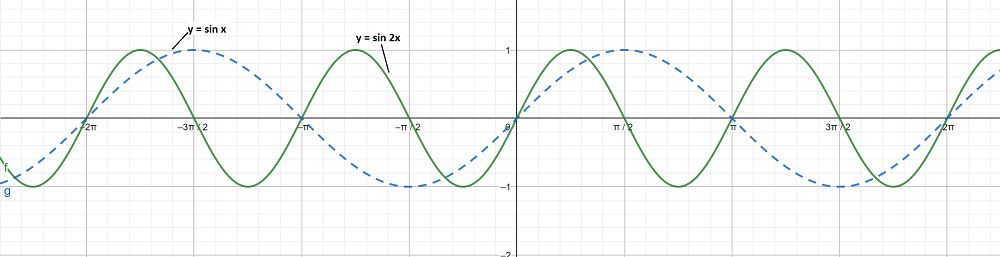The maximum and minimum values of y are 2 and -2 respectively.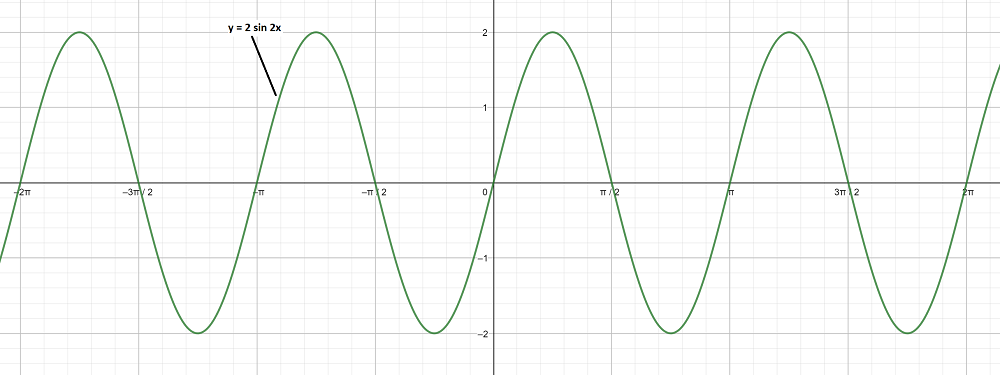### (ii) y = 3 sin x

Solution:

To obtain this graph y = 3 sin x,

First we draw the graph of y = sin x.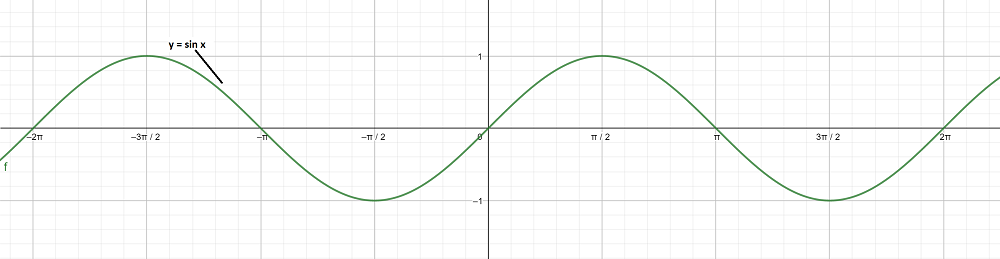The maximum and minimum values of y are 3 and -3 respectively.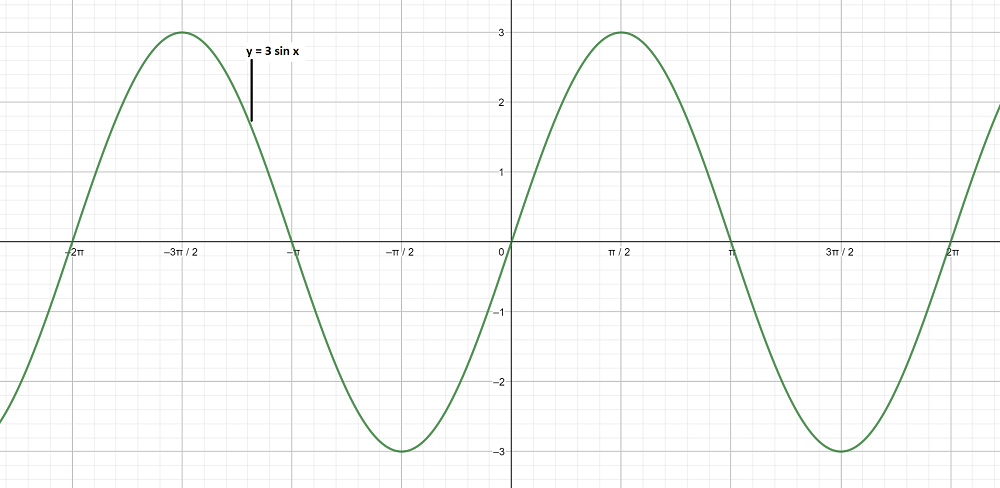### (iii)Solution:

To obtain this graph y-0 =,

On shifting the origin at (π/4, 0), we get

X =and Y = y – 0

On substituting these values, we get

Y = 2 sin X

First we draw the graph of Y = 2 sin X and shift it by π/4 to the right.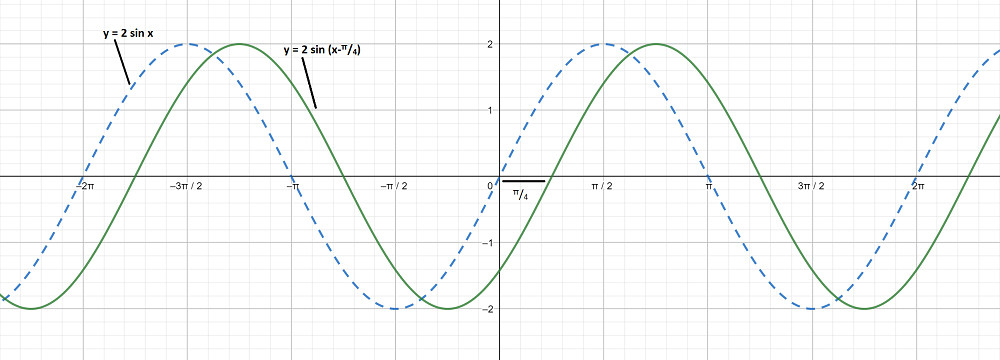### (iv) y = 2 sin (2x – 1)

Solution:

To obtain this graph y – 0 = 2 sin,

On shifting the origin at (1/2, 0), we get

X =and Y = y – 0

On substituting these values, we get

Y = 2 sin 2X

First we draw the graph of Y = 2 sin 2X and shift it by 1/2 to the right.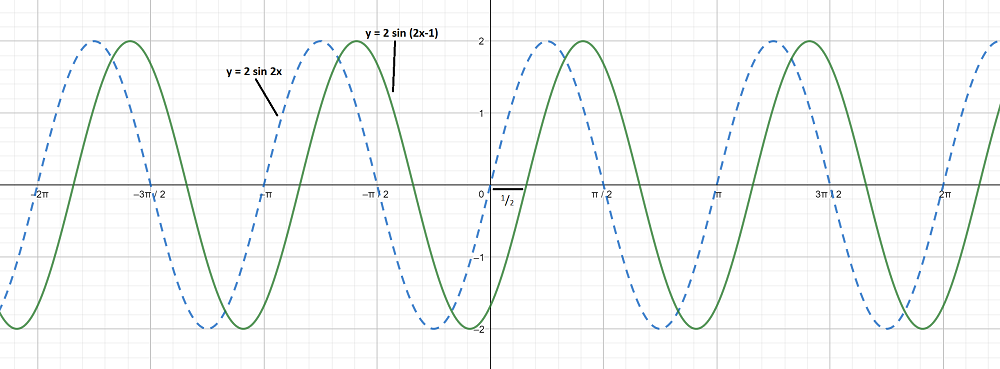### (v) y = 3 sin (3x + 1)

Solution:

To obtain this graph y – 0 = 3 sin 3,

On shifting the origin at(-1/3, 0), we get

X =and Y = y – 0

On substituting these values, we get

Y = 3 sin 3X

First we draw the graph of Y = 3 sin 3X and shift it by 1/3 to the left.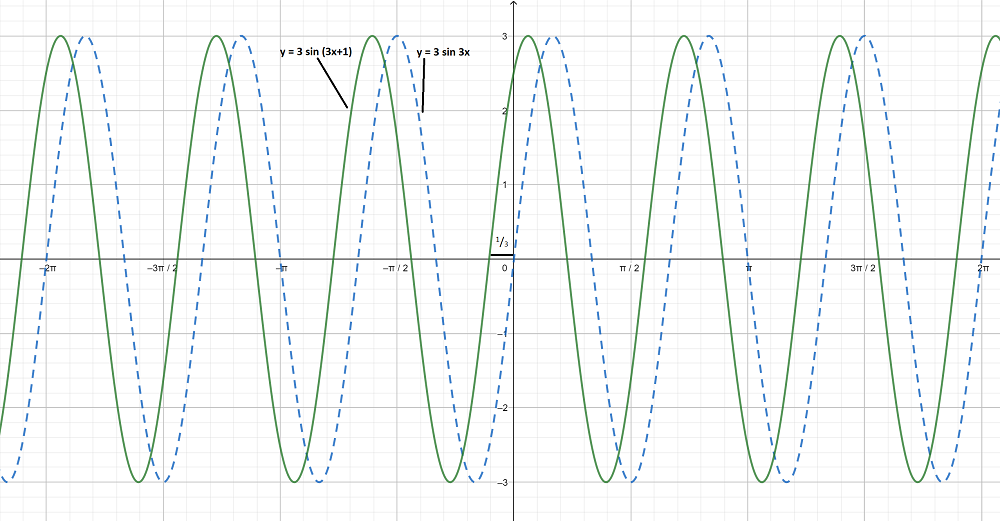### (vi)To obtain this graph y-0 =On shifting the origin at (π/8, 0), we get

X =and Y = y-0

On substituting these values, we get

Y = 3 sin 2X

First we draw the graph of Y = 3 sin 2X and shift it by π/8 to the right.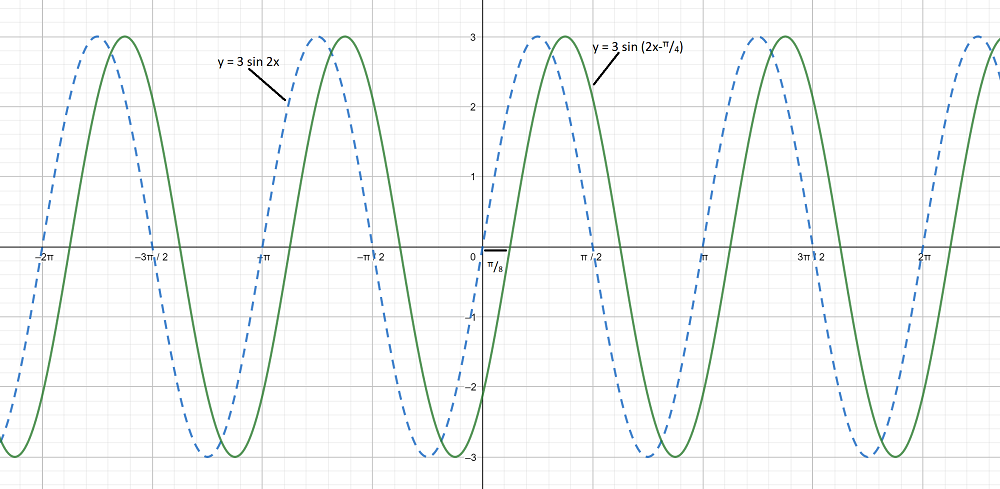### (i) y = sin x,Graph 1:

y = sin x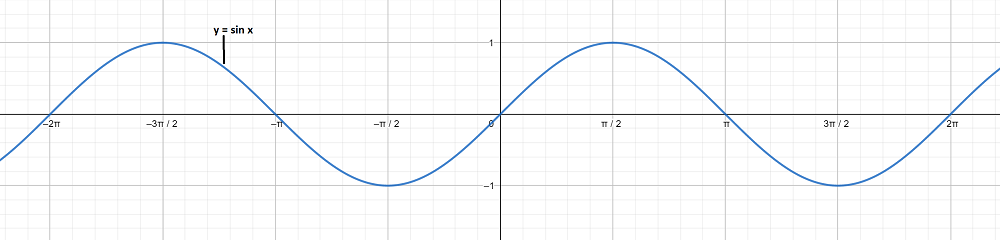Graph 2:

To obtain this graph y-0 =On shifting the origin at (-π/4, 0), we get

X =and Y = y – 0

On substituting these values, we get

Y = sin X

First we draw the graph of Y = sin X and shift it by π/4 to the left.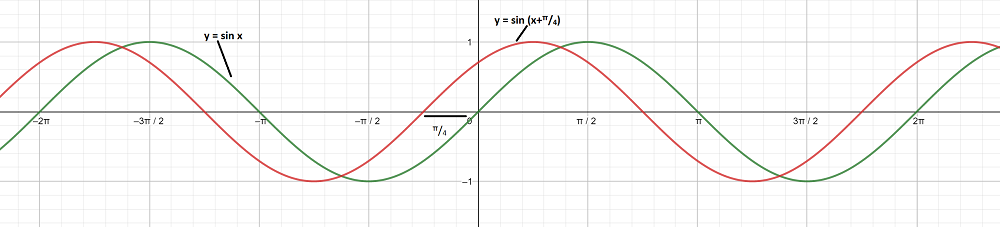The graph y = sin x andare on different axes are as follows: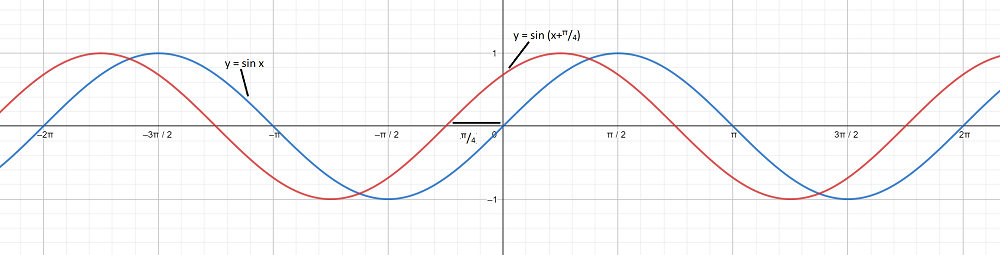### (ii) y = sin x, y = sin 3x

Graph 1:

y = sin x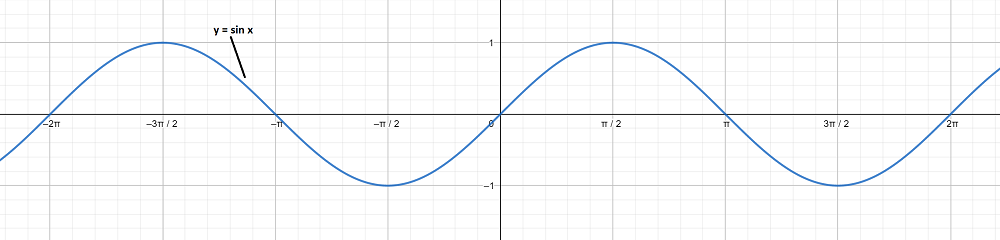Graph 2:

To obtain this graph y = sin 3x,

First we draw the graph of y = sin x and then divide the x-coordinates of the points where it crosses x-axis by 3.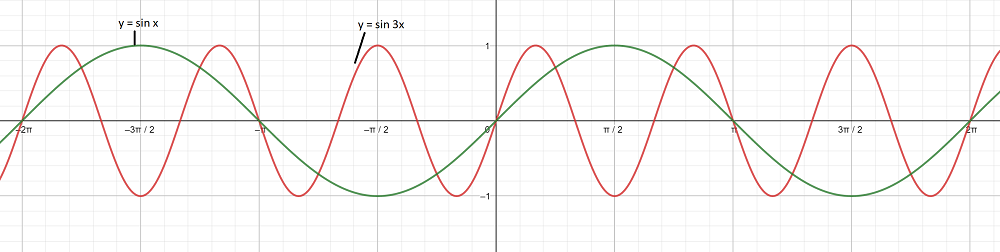The graph y = sin x and y = sin 3x are on different axes are as follows: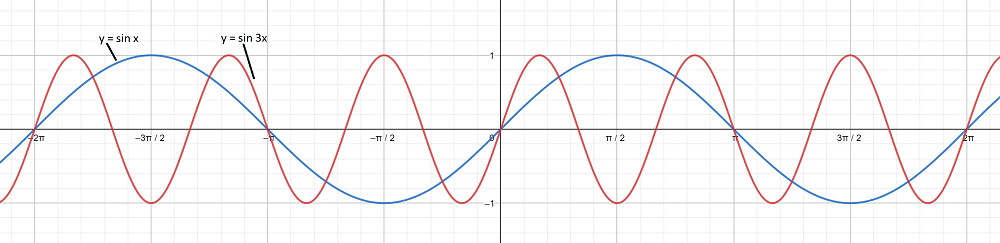My Personal Notes arrow_drop_up